# 7 Conclusion

In this article, we have performed a thorough linear and nonlinear analysis, from first principles, of electron acoustic excitations occurring in a nonthermal plasma consisting of hot-distributed electrons, adiabatic cool electrons, and immobile ions.

First, we have derived a linear dispersion relation, and investigated the dependence of the dispersion characteristics on the plasma environment (degree of suprathermality' through the parameter, plasma composition, and thermal effects).

Then, we have employed the Sagdeev pseudopotential method to investigate large amplitude localized nonlinear electrostatic structures (solitary waves), and to determine the region in parameter space where stationary profile solutions may exist. Only negative potential solitons were found. The existence domain for solitons was shown to become narrower in the range of solitary wave speed, with an increase in the excess of suprathermal electrons in the hot electron distribution (stronger suprathermality', lowervalue). The dependence of the soliton characteristics on the hot electron number density (through the parameter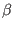) and on the hot-to-cool electron temperature ratio, were also studied. A series of appropriate examples of pseudopotential curves and soliton profiles were computed numerically, in order to confirm the predictions arising from the study of existence domains.

It may be added that ionic motion/inertia, here neglected, may also be included for a more accurate description, but is likely to have only minor quantitative effects.

We note, for completeness, that very recently two related papers have appeared with a scope apparently similar to that of the present article, viz., Refs. [52,39]. A word of comparison may therefore be appropriate here, for clarity. The latter authors  indicate that they are using a form of kappa distribution from one of the pioneering papers in the field.  Unfortunately, their expression for the characteristic speed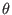does not agree with the standard expression , and thus the hot electron density does not take the usual form, Eq. (5).  Further, they use values of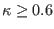, i.e., well below the standard-distribution cut-off of 3/2. Hence their results do not apply to the standard form ofdistribution. 

As regards the other paper,  it did not consider existence domains at all (apart from an indirect mention of an upper limit in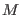, as commented on in Section 5 above). Further, no account is taken in the paper of the possible effects of Landau damping on sustainable nonlinear structures, and a number of the figures relate to values of(called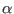in the paper) which lie in the unphysical, damped range.

We note that Ref.  has also carried out a “small amplitude" calculation yielding double layers. However, when evaluated numerically, these turn out to be well beyond the range of small amplitude, and that raises some doubts about the validity of the results (their Figures 8-10). Further, let us take together their Figures 2 and 8, and consider the case of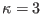and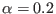(i.e., our). The latter is, of course, a value for which the linear wave is likely to be strongly Landau damped. It appears from the figures that a soliton occurs at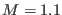with amplitude, while a double layer occurs for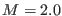with amplitude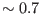. This combination of data does not satisfy the analytically-proven requirement that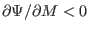, [48,49,50]. There is no obvious reason why that should be the case, and there thus seems to be an error in at least one of these two figures.

Finally, we point out that we have not sought double layers in our calculations. However, we would be surprised if they did occur, as they are usually found as the upper limit to a sequence of solitons for a polarity for which there is no other limit. In this case there clearly is an upper limit for negative solitary waves, arising from the constraint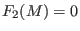. It is in principle possible for a double layer to occur at a lower value of, and be followed by larger amplitude solitons at higher, until the upper fluid cutoff such as a sonic point or an infinite compression cutoff is reached.  However, such behaviour depends on the the Sagdeev potential having a fairly complicated shape, with subsidiary local maxima, and we have not observed these for this model.

We are not aware of any experimental studies with which these theoretical results may be directly compared. However, it has previously been shown that wave data may be used to obtain an estimate for, thus acting as a diagnostic for the distribution function [19,55].

Similarly, in this case there are a number of indicators amongst our results which experimenters may wish to consider when interpreting observations. Thus, for instance, a lower normalized phase velocity of the linear electron-acoustic wave than would be predicted by a Maxwellian model (see Fig. 1) could be used to evaluate.

Secondly, from Fig. 2 one sees that in low-plasmas the range of normalized soliton speeds is both narrower and of larger value than one would expect for a Maxwellian. Thus, if solitons are found with normalized speeds around, these can be understood only by allowing for additional suprathermal electrons (lower). Further, from Fig. 2 it follows that Maxwellian electrons give rise to a cutoff in the density ratio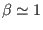, and hence solitons observed in such plasmas can only be explained in terms of lower.

From Fig. 5 we note that at fixed values of the normalized soliton speed,, the amplitudes of the perturbations of the normalized potential, cool electron density and cool electron speed due to the solitary waves all increase with decreasing. This is related to the increase of the true Mach numberfor smaller, as the phase velocity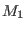is decreased. Thus larger disturbances are likely to be associated with increased suprathermality.

Finally, turning to Fig. 6, two effects are observed: At fixed true Mach number,, the soliton amplitude decreases with decreasing(increasing suprathermality). Despite that, the maximum values of soliton amplitude is found to occur not for a Maxwellian, but for the relatively low-values of around 2.5-3. Thus, again, large observed amplitudes are likely to be associated with a low-plasma.

Hence, as shown above, these results could assist in the understanding of solitary waves observed in two-temperature space plasmas, which are often characterized by a suprathermal electron distribution.

Ashkbiz Danehkar
2018-03-28